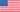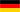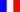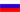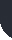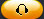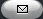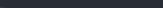Task Management Software for Team Collaboration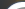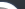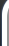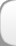HomeDownloadSupportPurchase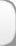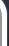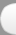Installation GuideStartUp GuideOnline Help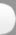# Project Budget Factor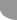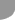Home  » Products  » VIP Task Manager Pro  » Tutorials  » Customization Guide  » Project Budget Factor

Project Budget Factor

Project performance is measured according to three project baselines: cost, schedule and scope. The given KPI refers to cost baseline or budget spread over time which measures project cost performance. The usage of Project Budget Factor as a key performance indicator is stipulated by the nature of your project. Project Budget Factor KPI is widely used for measuring the progress of construction projects and the contractor performance in particular. This indicator shows whether the project is on budget after project closure comparing with its baseline cost.

To compute Project Budget Factor the following formula is used:

Project Budget Factor = Actual Cost / (Baseline Cost + Approved Changes Cost)

For construction projects Actual Cost is the sum of three items: Labor Costs, Cost of Materials and Other Expenses. Other Expenses include Haulage Costs (expenses related to hauling materials, supplies, equipment, etc.) and other costs to perform constructions operations.
The formula for calculating Actual Cost may look like this:

Actual Cost = (Actual Work * Standard Rate) + (Actual Overtime Work * Overtime Rate) + Resource per Use Costs + Task Fixed Cost = (Actual Work * Standard Rate) + (Actual Overtime Work * Overtime Rate) + Resource per Use Costs + Cost of Materials + Other Expenses.

Baseline Cost is estimated total cost authorized during planning stage of a project.
Baseline is calculated by similar formula above but using baseline values:

Baseline Cost = (Work * Standard Rate) + (Overtime Work * Overtime Rate) + Resource per Use Cost + Task Fixed Cost = (Work * Standard Rate) + (Overtime Work * Overtime Rate) + Resource per Use Cost + Cost of Materials + Other Expenses
Approved Changes Cost includes the cost of all authorized changes from pre-design stage to project closure. It can be change request for additional staff member or request to defer due date.

If the value of this KPI is less than 1, the project is on track by cost. If the value is greater than 1 the project cost was exceeded comparing with cost baseline.

To calculate Project Budget Factor follow the steps below:

If cost should include labor cost and cost for materials the formula may look like this:
ToFloat(\$cf_overtime_work)*24 * \$cf_overtime_rate + \$cf_resource_per_use_cost + \$cf_cost_of_materials + \$cf_other_expenses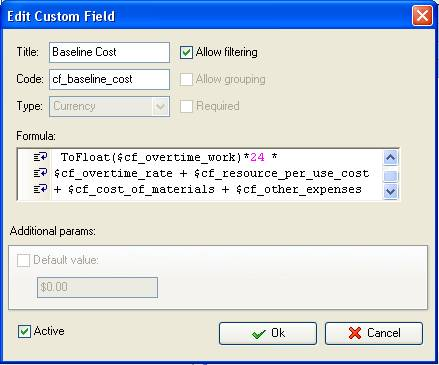• Add the custom field “Actual Cost” with code “cf_actual_cost”

Following the formula for Baseline Cost the formula for Actual Cost may be as follows:

ToFloat(\$cf_actual_work)*24 * \$cf_standard_rate + ToFloat(\$cf_actual_overtime_work)*24 * \$cf_overtime_rate + \$cf_resource_per_use_cost + \$cf_cost_of_materials + \$cf_other_expenses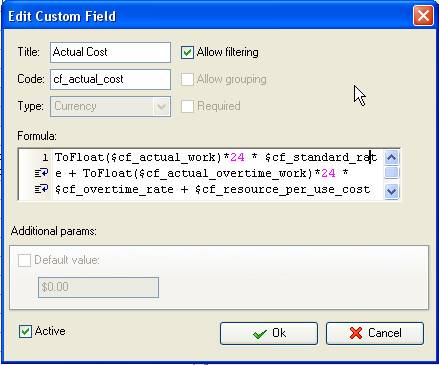• Add custom field “Approved Changes Cost” with code “cf_approved_changes_cost”: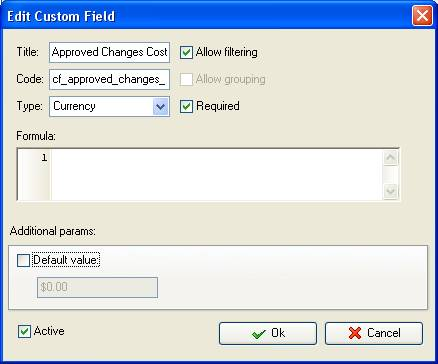• Add the custom field “Budget Factor” with Type “Float” and the formula:

\$cf_actual_cost / (\$cf_baseline_cost + \$cf_approved_changes_cost)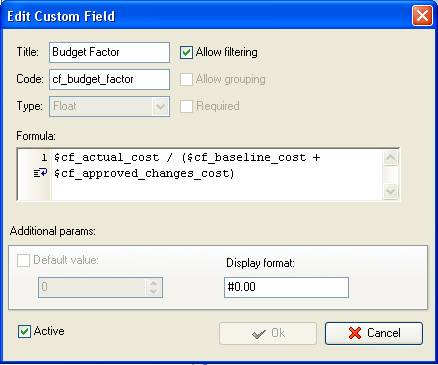On Task Tree select the task group for which you need to display Project Budget Factor and select “Custom fields options”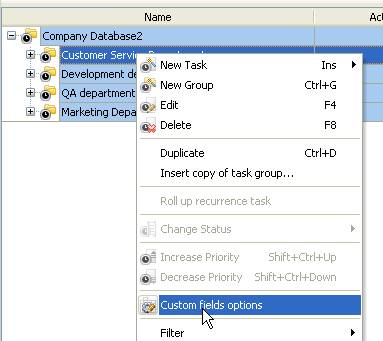• Set the following custom fields settings for the custom fields: “Baseline Cost”, “Actual Cost”, and “Budget Factor”

- In “Settings for” select “This task group”
- select “Enable”
- select “Calculated by custom field formula (for task group)”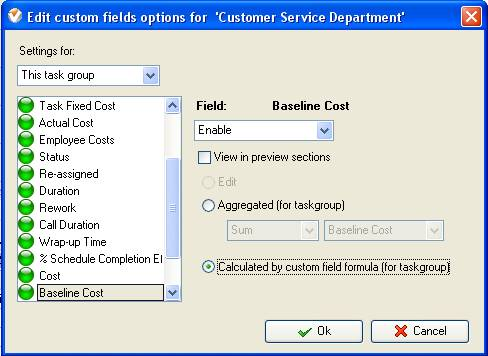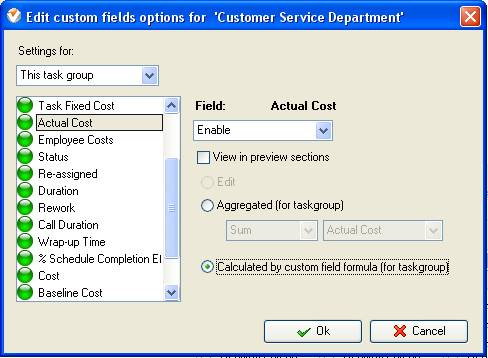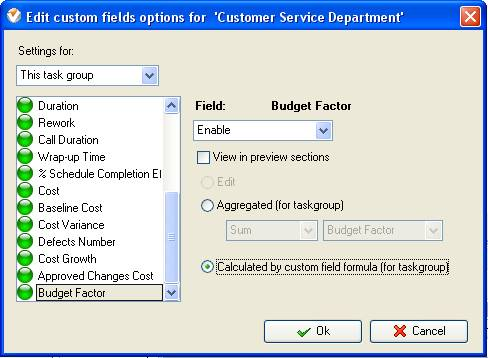• To enter the value of the custom field “Approved Changes Cost” you need to right click on task group with project tasks, select “Custom fields options” and select “Edit”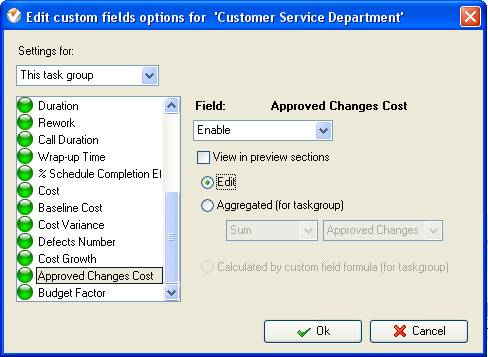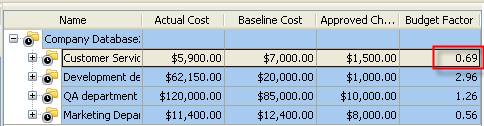Project Budget Factor on Task Tree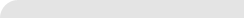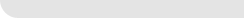» VIP Task Manager Pro VIP Task Manager Std Testimonials Turnkey Solution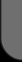ProductsSolutionsDownloadSupportPurchaseContactsForumVideoMy Account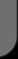Copyright © 2004 - 2023 Task Management Software